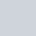in

# Oracle Apex: Loop Through Interactive Grid Records

The following are some examples to show you how to loop through interactive grid records in Oracle Apex.

To get interactive grid records using the JavaScript, first, we need to specify the Static ID for the interactive grid report. To do this, click on your interactive grid and in the Advanced section, specify the Static ID as shown in the below image, I defined "igemp":Now you have to choose the event on which you want to read all rows by looping through the interactive grid records. For example, you can create a dynamic action on any of the columns of an interactive grid and select the event Get Focus or Lose Focus, etc.

To demonstrate this example, I have used a button and created a dynamic action on a button click event to execute JavaScript code. And on click of the button, I will loop through all the records of the interactive grid and will print the console log.

The following is an example in which I will loop through all the records of the interactive grid and will print the column value of FRIST_NAME:

## Example-1: Loop Through All Records and Print a Column Value

```var model = apex.region("igemp").widget().interactiveGrid("getViews", "grid").model;
model.forEach(function(igrow) {
console.log(igrow[model.getFieldKey("FIRST_NAME")]);
});```

#### Output:## Example-2: Loop Through Interactive Grid Records and Get the Column Value in a Variable

In the following example, it will get the FIRST_NAME and LAST_NAME columns value in variables, and then it will print on the console log.

```var model = apex.region("igemp").widget().interactiveGrid("getViews", "grid").model;
var v_fname, v_lname;
v_fname = model.getFieldKey("FIRST_NAME");
v_lname = model.getFieldKey("LAST_NAME");
model.forEach(function(igrow) {
console.log(igrow[v_fname] +" "+ igrow[v_lname]);
});```

#### Output:

```Neena Kochhar Lex De Haan Valliru Pataballa Alexanderia Hunold Bruce Ernst```

## Example-3: Loop Through Records and Sum a Column Value

In the following example, it will calculate the total of the SALARY column and then will print on the console log.

```var model = apex.region("igemp").widget().interactiveGrid("getViews", "grid").model;
var n_sal, n_totsal = 0;
col_salary = model.getFieldKey("SALARY");
model.forEach(function(igrow) {
n_sal = parseInt(igrow[col_salary], 10);
if (!isNaN(n_sal)) {
n_totsal += n_sal;
}
});
console.log(n_totsal);```

You can get the values in an item on your page in Oracle Apex. For example, add the below JavaScript code line to the bottom of the above code to get the total salary value in an item P2_TOTSAL:

`\$s('P2_TOTSAL', n_totsal);`

#### Related Tutorials: